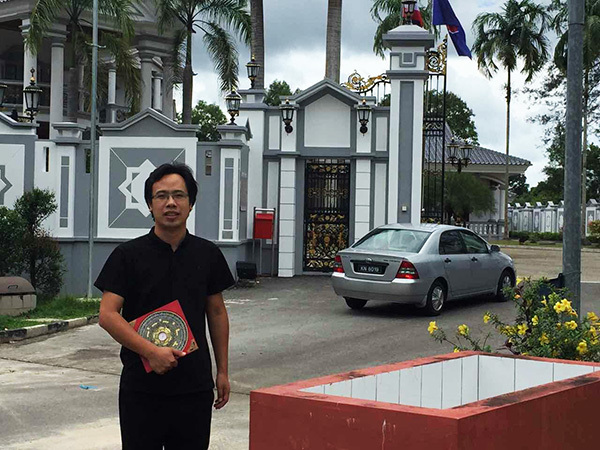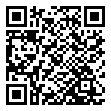|

# 陈益峰谈住宅户型的常见风水问题

风水是自然与人之间的作用关系，是人类生活的守护者。陈益峰师傅认为现实中，完全满足风水理论上的房子很少见，不是这块有点毛病，或是那块有些不利，只是存在毛病大小的问题。这从侧面也反应出，不管是富人家还是穷人家，都没有一个家庭是十全十美的。风水上十全十美的房子，只能停留在理论上，这也是符合宇宙发展规律的。大自然的发展变化规律是：没有最好，只有寻找相对的更好。陈益峰师傅是当代最有成就的风水名家之一，其高超的风水技术与专业的风水精神，得到国内外众多风水研究者的高度赞扬，先后前往德国柏林、法兰克福、波兰华沙、拉多姆、澳大利亚、新加坡、马来西亚、文莱等国家相宅相地，传播风水学说，在国际上有很高的知名度。陈师傅认为现代住宅的户型格局有相似之处，一般风水需要注意的问题也有一些相似。

1、户型不正，不安定

风水中户型是非常重要的，它就象一个人长的面相一样，表现出内在的信息。一个长得凶神恶煞一样的人，他的体内一定是邪气场太重。天地间都讲究正气，因此我们在选房子时一定要尽量选择方正一点的户型，不管是正方形还是长方形。千万不要选择那些三角形的、l型的、u型的、十字型的等各种不正房型。这对居住的人影响是非常大的，时间长了会影响人的思维，进而影响到人的情绪。2、房屋缺角，多伤人

房屋缺角也比较常见，房子是一个整体，从易学上讲是分八卦九宫的，每个方位都代表不同的寓意，就象人体的五脏六腑一样，每个器官都有自己的作用。房屋缺角就象人体缺少器官一样，于居住其中的主人不利。比如缺西北角就于男主人和事业不利，一是影响到男主人的身体和事业，二还会影响到家庭的婚姻问题。

3、地基潮湿不干净，败家

住宅地基最好是干燥的耕地或者山地，最差的是水沟、垃圾场、砖窑、坟场等位置，地基不干净，久住家人容易生病。如果住宅架在水沟或者河流、道路、地铁上面，久住家人容易败家，美国的流水别墅，就是将住宅架在山沟上，看似很美丽，实际上业主是败家破产了的，后来基本上无人敢住，只能改建为博物馆。3、宅大人少，家不旺

《皇帝宅经》中讲宅有五虚，其中一点就是指宅大人少。就是说现在不少有钱的人家中本来就二三口人，正常是有一百多平米就可以了，可是却非得买上几百平米的大房子，不是楼中楼就是别墅。我们并不是忌妒人家有这么大的房子，只是从风水的角度来说明一下大房子的不利之处而矣。如果人多住还可以，人少就不行了，宅过大气场就会过旺，人少人气就不旺，人的气场制不住宅子的气场，这就属于宅欺人了。说白了就是人压不宅，因此居住在其中的人就会不顺，运气不旺等。

5、中间厕所，伤心脏

有的房子设计时不知是出于哪方面的原因，把卫生间设计在了房子的中间，这种格局也很常见。风水上讲房子的中收叫做宅心，就犹如人的中心处的心脏一样，是非常重要的一个位置，这个位置宜空，宜静，不喜有高大的东西压在这里。卫生间是污浊之气，还每天有人进出，并放下水等，这就严重地影响了宅心的气场，因此不好的气场在这引动一定会带来不好的结果。同时卫生间设在西南角和西北角对男女主人也是不利的。

6、西北厨房，多短寿

如果是入户门在西侧，一般的房子设计都是在进入入户门后的左手侧是厨房，这样的格局比比皆是，这样的话那么厨房就在西北角了，那些搞户型设计的人员，几乎是没有一个人能懂风水知识的，象乾位家庭最重要的位置，不少户型设计中就是厨房在这个位置，这哪能行呢。在五行中乾卦属于金，而厨房是属于火的，火正好克金，这不是抑制男人的事业发展嘛，使其事业受挫，处处不顺。经过实践总结有三分之一男人的事业不顺，与这个有关。

7、东南厨房，出人才

炉灶为什么重要，就是因为其火力十足，阳气十足，风水影响极大，一般位于住宅的东侧比西侧旺。最好的位置是住宅的东南角有炉灶，后人必出惊世之人才。东南角主孩子的文事，东南角见厨房，孩子脑瓜聪明，喜欢学习专研，爱动脑经，尤其是利于女孩子，如果孩子是龙蛇猪狗的属相，最好了，住这种厨房的房子，百分之九十九都是有出息的人。厨房的位置在东面或者东南角，这叫烧东不烧西，烧东出贵子，烧西出蠢货。厨房在东面，儿子最容易有出息，出大学生的概率极高，东方为长男震卦，主事业、官运、贵人，东方见厨房，阳气十足，儿子有富有贵。8、门窗过多，不聚气

有很多的阳宅泄气过多，风水上讲藏风聚气，只有气聚在一起了，才会起好运势。若是藏不住气，那自然也就不能藏风聚气了。其中有一种情况就是居家的门和窗户过多，尤其是住宅的大门与客厅的窗户直对的时候，泄气更厉害。窗是泄气口，门和窗户过多就存不住气，从入户门进来的气直接就从窗户上泄出去了，因此也是达不到藏风聚气的目地。室内泄气过多直接会导致主人的身体不好，这样的例子我们遇到了不少，还有就是家中存不住钱，进来的钱财总会因各种事花出去。

9、一剑穿堂，不聚财

一剑穿堂基本上是由三个元素构成，一是入户门，二是客厅，三是阳台。也就是说这三个成一条直线，具体地说就是当你一打开入户门时首先看到的是客厅，在往前看就直接能看到阳台，这就是犯一剑穿堂的格局。就象一把利剑直穿客厅(也叫大堂)，因此称为一剑穿堂。这种格局不好的原因是房子不聚气。风水学的核心就是讲究藏风聚气，只有存住气才能旺财运、事业等等。而这种格局则属于泄气的格局，气由门进来之后一定要存住，客厅就相当于葫芦肚一样，具有存气的功能。可是如果客厅在与门相对的地方直接对着阳台，那由入户门进来的吉气就直接从阳台上泄掉了。家中存不住气哪能旺起来，这就是家中事业不旺的原因。（记者/杨启琪）

`声明：本文由入驻焦点开放平台的作者撰写，除焦点官方账号外，观点仅代表作者本人，不代表焦点立场错误信息举报电话： 400-099-0099，邮箱：jubao@vip.sohu.com，或点此进行意见反馈，或点此进行举报投诉。`A B C D E F G H J K L M N P Q R S T W X Y Z
A - B - C - D - E
• A
• 鞍山
• 安庆
• 安阳
• 安顺
• 安康
• 澳门
• B
• 北京
• 保定
• 包头
• 巴彦淖尔
• 本溪
• 蚌埠
• 亳州
• 滨州
• 北海
• 百色
• 巴中
• 毕节
• 保山
• 宝鸡
• 白银
• 巴州
• C
• 承德
• 沧州
• 长治
• 赤峰
• 朝阳
• 长春
• 常州
• 滁州
• 池州
• 长沙
• 常德
• 郴州
• 潮州
• 崇左
• 重庆
• 成都
• 楚雄
• 昌都
• 慈溪
• 常熟
• D
• 大同
• 大连
• 丹东
• 大庆
• 东营
• 德州
• 东莞
• 德阳
• 达州
• 大理
• 德宏
• 定西
• 儋州
• 东平
• E
• 鄂尔多斯
• 鄂州
• 恩施
F - G - H - I - J
• F
• 抚顺
• 阜新
• 阜阳
• 福州
• 抚州
• 佛山
• 防城港
• G
• 赣州
• 广州
• 桂林
• 贵港
• 广元
• 广安
• 贵阳
• 固原
• H
• 邯郸
• 衡水
• 呼和浩特
• 呼伦贝尔
• 葫芦岛
• 哈尔滨
• 黑河
• 淮安
• 杭州
• 湖州
• 合肥
• 淮南
• 淮北
• 黄山
• 菏泽
• 鹤壁
• 黄石
• 黄冈
• 衡阳
• 怀化
• 惠州
• 河源
• 贺州
• 河池
• 海口
• 红河
• 汉中
• 海东
• 怀来
• I
• J
• 晋中
• 锦州
• 吉林
• 鸡西
• 佳木斯
• 嘉兴
• 金华
• 景德镇
• 九江
• 吉安
• 济南
• 济宁
• 焦作
• 荆门
• 荆州
• 江门
• 揭阳
• 金昌
• 酒泉
• 嘉峪关
K - L - M - N - P
• K
• 开封
• 昆明
• 昆山
• L
• 廊坊
• 临汾
• 辽阳
• 连云港
• 丽水
• 六安
• 龙岩
• 莱芜
• 临沂
• 聊城
• 洛阳
• 漯河
• 娄底
• 柳州
• 来宾
• 泸州
• 乐山
• 六盘水
• 丽江
• 临沧
• 拉萨
• 林芝
• 兰州
• 陇南
• M
• 牡丹江
• 马鞍山
• 茂名
• 梅州
• 绵阳
• 眉山
• N
• 南京
• 南通
• 宁波
• 南平
• 宁德
• 南昌
• 南阳
• 南宁
• 内江
• 南充
• P
• 盘锦
• 莆田
• 平顶山
• 濮阳
• 攀枝花
• 普洱
• 平凉
Q - R - S - T - W
• Q
• 秦皇岛
• 齐齐哈尔
• 衢州
• 泉州
• 青岛
• 清远
• 钦州
• 黔南
• 曲靖
• 庆阳
• R
• 日照
• 日喀则
• S
• 石家庄
• 沈阳
• 双鸭山
• 绥化
• 上海
• 苏州
• 宿迁
• 绍兴
• 宿州
• 三明
• 上饶
• 三门峡
• 商丘
• 十堰
• 随州
• 邵阳
• 韶关
• 深圳
• 汕头
• 汕尾
• 三亚
• 三沙
• 遂宁
• 山南
• 商洛
• 石嘴山
• T
• 天津
• 唐山
• 太原
• 通辽
• 铁岭
• 泰州
• 台州
• 铜陵
• 泰安
• 铜仁
• 铜川
• 天水
• 天门
• W
• 乌海
• 乌兰察布
• 无锡
• 温州
• 芜湖
• 潍坊
• 威海
• 武汉
• 梧州
• 渭南
• 武威
• 吴忠
• 乌鲁木齐
X - Y - Z
• X
• 邢台
• 徐州
• 宣城
• 厦门
• 新乡
• 许昌
• 信阳
• 襄阳
• 孝感
• 咸宁
• 湘潭
• 湘西
• 西双版纳
• 西安
• 咸阳
• 西宁
• 仙桃
• 西昌
• Y
• 运城
• 营口
• 盐城
• 扬州
• 鹰潭
• 宜春
• 烟台
• 宜昌
• 岳阳
• 益阳
• 永州
• 阳江
• 云浮
• 玉林
• 宜宾
• 雅安
• 玉溪
• 延安
• 榆林
• 银川
• Z
• 张家口
• 镇江
• 舟山
• 漳州
• 淄博
• 枣庄
• 郑州
• 周口
• 驻马店
• 株洲
• 张家界
• 珠海
• 湛江
• 肇庆
• 中山
• 自贡
• 资阳
• 遵义
• 昭通
• 张掖
• 中卫

1室1厅1厨1卫1阳台

1
2
3
4
5

0
1
2

1

1

0
1
2
3报名成功，资料已提交审核A B C D E F G H J K L M N P Q R S T W X Y Z
A - B - C - D - E
• A
• 鞍山
• 安庆
• 安阳
• 安顺
• 安康
• 澳门
• B
• 北京
• 保定
• 包头
• 巴彦淖尔
• 本溪
• 蚌埠
• 亳州
• 滨州
• 北海
• 百色
• 巴中
• 毕节
• 保山
• 宝鸡
• 白银
• 巴州
• C
• 承德
• 沧州
• 长治
• 赤峰
• 朝阳
• 长春
• 常州
• 滁州
• 池州
• 长沙
• 常德
• 郴州
• 潮州
• 崇左
• 重庆
• 成都
• 楚雄
• 昌都
• 慈溪
• 常熟
• D
• 大同
• 大连
• 丹东
• 大庆
• 东营
• 德州
• 东莞
• 德阳
• 达州
• 大理
• 德宏
• 定西
• 儋州
• 东平
• E
• 鄂尔多斯
• 鄂州
• 恩施
F - G - H - I - J
• F
• 抚顺
• 阜新
• 阜阳
• 福州
• 抚州
• 佛山
• 防城港
• G
• 赣州
• 广州
• 桂林
• 贵港
• 广元
• 广安
• 贵阳
• 固原
• H
• 邯郸
• 衡水
• 呼和浩特
• 呼伦贝尔
• 葫芦岛
• 哈尔滨
• 黑河
• 淮安
• 杭州
• 湖州
• 合肥
• 淮南
• 淮北
• 黄山
• 菏泽
• 鹤壁
• 黄石
• 黄冈
• 衡阳
• 怀化
• 惠州
• 河源
• 贺州
• 河池
• 海口
• 红河
• 汉中
• 海东
• 怀来
• I
• J
• 晋中
• 锦州
• 吉林
• 鸡西
• 佳木斯
• 嘉兴
• 金华
• 景德镇
• 九江
• 吉安
• 济南
• 济宁
• 焦作
• 荆门
• 荆州
• 江门
• 揭阳
• 金昌
• 酒泉
• 嘉峪关
K - L - M - N - P
• K
• 开封
• 昆明
• 昆山
• L
• 廊坊
• 临汾
• 辽阳
• 连云港
• 丽水
• 六安
• 龙岩
• 莱芜
• 临沂
• 聊城
• 洛阳
• 漯河
• 娄底
• 柳州
• 来宾
• 泸州
• 乐山
• 六盘水
• 丽江
• 临沧
• 拉萨
• 林芝
• 兰州
• 陇南
• M
• 牡丹江
• 马鞍山
• 茂名
• 梅州
• 绵阳
• 眉山
• N
• 南京
• 南通
• 宁波
• 南平
• 宁德
• 南昌
• 南阳
• 南宁
• 内江
• 南充
• P
• 盘锦
• 莆田
• 平顶山
• 濮阳
• 攀枝花
• 普洱
• 平凉
Q - R - S - T - W
• Q
• 秦皇岛
• 齐齐哈尔
• 衢州
• 泉州
• 青岛
• 清远
• 钦州
• 黔南
• 曲靖
• 庆阳
• R
• 日照
• 日喀则
• S
• 石家庄
• 沈阳
• 双鸭山
• 绥化
• 上海
• 苏州
• 宿迁
• 绍兴
• 宿州
• 三明
• 上饶
• 三门峡
• 商丘
• 十堰
• 随州
• 邵阳
• 韶关
• 深圳
• 汕头
• 汕尾
• 三亚
• 三沙
• 遂宁
• 山南
• 商洛
• 石嘴山
• T
• 天津
• 唐山
• 太原
• 通辽
• 铁岭
• 泰州
• 台州
• 铜陵
• 泰安
• 铜仁
• 铜川
• 天水
• 天门
• W
• 乌海
• 乌兰察布
• 无锡
• 温州
• 芜湖
• 潍坊
• 威海
• 武汉
• 梧州
• 渭南
• 武威
• 吴忠
• 乌鲁木齐
X - Y - Z
• X
• 邢台
• 徐州
• 宣城
• 厦门
• 新乡
• 许昌
• 信阳
• 襄阳
• 孝感
• 咸宁
• 湘潭
• 湘西
• 西双版纳
• 西安
• 咸阳
• 西宁
• 仙桃
• 西昌
• Y
• 运城
• 营口
• 盐城
• 扬州
• 鹰潭
• 宜春
• 烟台
• 宜昌
• 岳阳
• 益阳
• 永州
• 阳江
• 云浮
• 玉林
• 宜宾
• 雅安
• 玉溪
• 延安
• 榆林
• 银川
• Z
• 张家口
• 镇江
• 舟山
• 漳州
• 淄博
• 枣庄
• 郑州
• 周口
• 驻马店
• 株洲
• 张家界
• 珠海
• 湛江
• 肇庆
• 中山
• 自贡
• 资阳
• 遵义
• 昭通
• 张掖
• 中卫• 手机• 分享
• 设计
免费设计
• 计算器
装修计算器
• 入驻
合作入驻
• 联系
联系我们
• 置顶
返回顶部